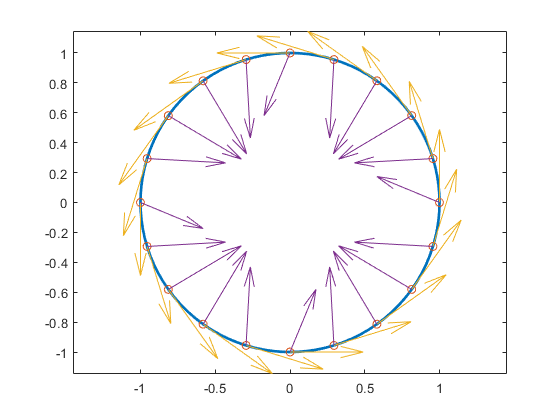# fntlr

Taylor coefficients

## Syntax

```taylor = fntlr(f,dorder,x) ```

## Description

`taylor = fntlr(f,dorder,x) ` returns the nonnormalized Taylor coefficients, up to the given order `dorder` and at the given `x`, of the function described in `f` .

For a univariate function and a scalar `x`, this is the vector

`$T\left(f,\text{dorder},x\right):=\left[f\left(x\right);Df\left(x\right);...;{D}^{\text{dorder}-1}f\left(x\right)\right]$`

If, more generally, the function in `f` is `d`-valued with `d>1` or even `prod(d)>1` and/or is `m`-variate for some `m>1`, then `dorder` is expected to be an `m`-vector of positive integers, `x` is expected to be a matrix with `m` rows, and, in that case, the output is of size `[prod(d)*prod(dorder),size(x,2)]`, with its j-th column containing

`$T\left(f,\text{dorder},x\left(:,j\right)\right)\left(i1,...,im\right)={D}_{1}{}^{i1-1}...{D}_{m}{}^{im-1}f\left(x\left(:,j\right)\right)$`

for `i1=1:dorder(1)`, ..., `im=1:dorder(m)`. Here, Dif is the partial derivative of f with respect to its ith argument.

## Examples

If `f` contains a univariate function and `x` is a scalar or a 1-row matrix, then `fntlr(f,3,x)` produces the same output as the statements

```df = fnder(f); [fnval(f,x); fnval(df,x); fnval(fnder(df),x)]; ```

As a more complicated example, look at the Taylor vectors of order 3 at 21 equally spaced points for the rational spline whose graph is the unit circle:

```ci = rsmak('circle'); in = fnbrk(ci,'interv'); t = linspace(in(1),in(2),21); t(end)=[]; v = fntlr(ci,3,t); ```

We plot `ci `along with the points `v(1:2,:)`, to verify that these are, indeed, points on the unit circle.

```fnplt(ci), hold on, plot(v(1,:),v(2,:),'o') ```

Next, to verify that `v(3:4,j)` is a vector tangent to the circle at the point `v(1:2,j)`, we use the MATLAB® `quiver` command to add the corresponding arrows to our plot:

```quiver(v(1,:),v(2,:),v(3,:),v(4,:)) ```

Finally, what about `v(5:6,:)`? These are second derivatives, and we add the corresponding arrows by the following `quiver` command, thus finishing First and Second Derivative of a Rational Spline Giving a Circle.

```quiver(v(1,:),v(2,:),v(5,:),v(6,:)), axis equal, hold off ```

First and Second Derivative of a Rational Spline Giving a CircleNow, our curve being a circle, you might have expected the 2nd derivative arrows to point straight to the center of that circle, and that would have been indeed the case if the function in `ci` had been using arclength as its independent variable. Since the parameter used is not arclength, we use the formula, given in Example: B-form Spline Approximation to a Circle, to compute the curvature of the curve given by `ci` at these selected points. For ease of comparison, we switch over to the variables used there and then simply use the commands from there.

```dspt = v(3:4,:); ddspt = v(5:6,:); kappa = abs(dspt(1,:).*ddspt(2,:)-dspt(2,:).*ddspt(1,:))./... (sum(dspt.^2)).^(3/2); max(abs(kappa-1)) ans = 2.2204e-016 ```

The numerical answer is reassuring: at all the points tested, the curvature is 1 to within roundoff.